Conge 精进

# AI 笔记 Week 06 Probability

2017-10-12

Week 6 Announcement

This week you should watch Lesson 5, Probability, and read Chapter 13 in AIMA (Russell & Norvig). Assignment 3:  Bayes Nets Sampling

# Challenge question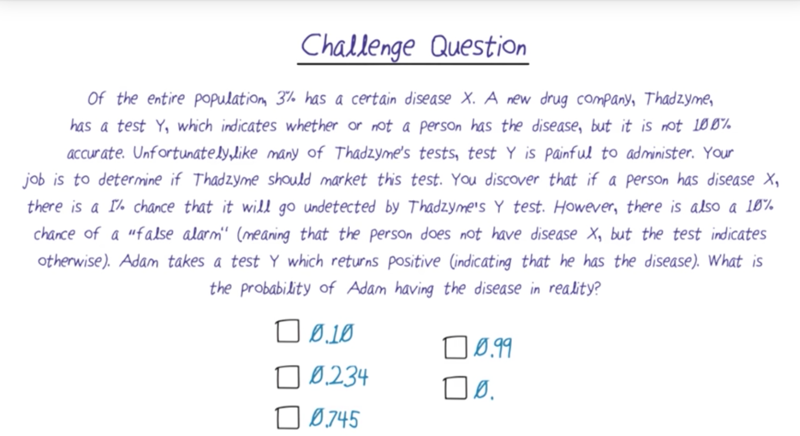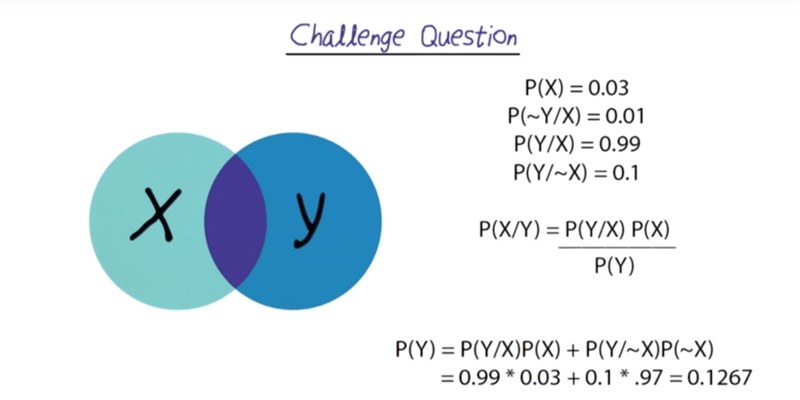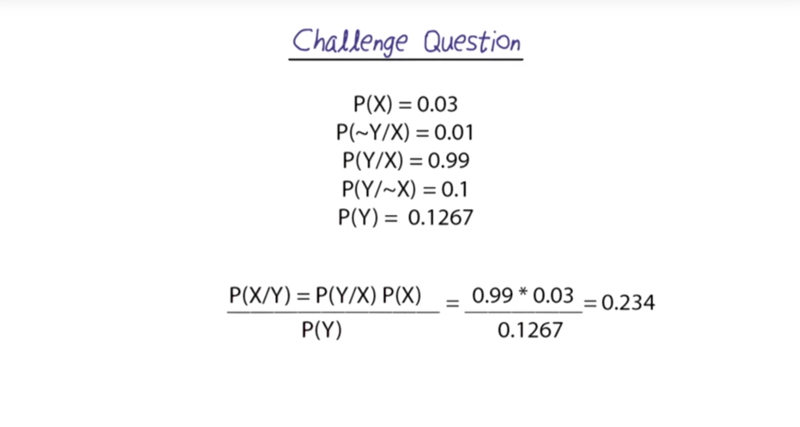• P(x) is the probability of the disease without other constraints
•  P(Y) is calculated P(Y ~X)P(~X) + P(Y X)P(X)

# Intro To Probability And Bayes Nets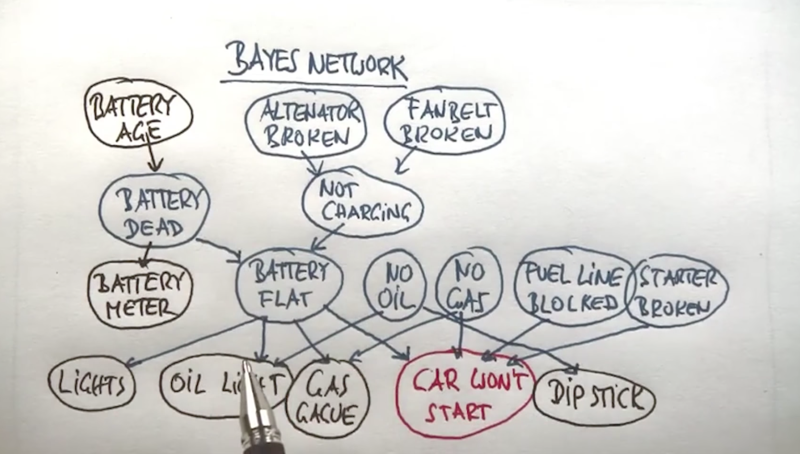• in the above example, we have random variables represents events which are connected by arrows to describe the relationships.
• the arrows indicate that the child nodes are influenced by their parents, and the influence can be a deterministic or probabilistic way.
• Bayes net is a compact representation of the distribution of the large probability distribution of all the variables.
• With Bayes net, we can specify the distribution, observe certain variables and compute probabilities of unobserved variables.

## outline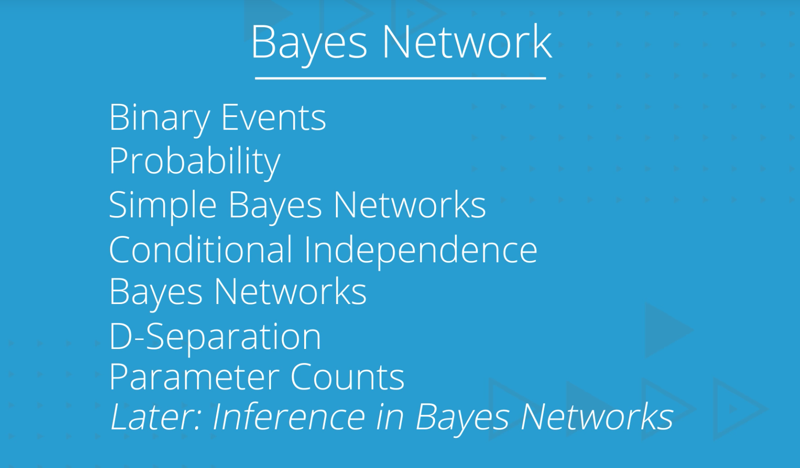# Probability / Coin Flip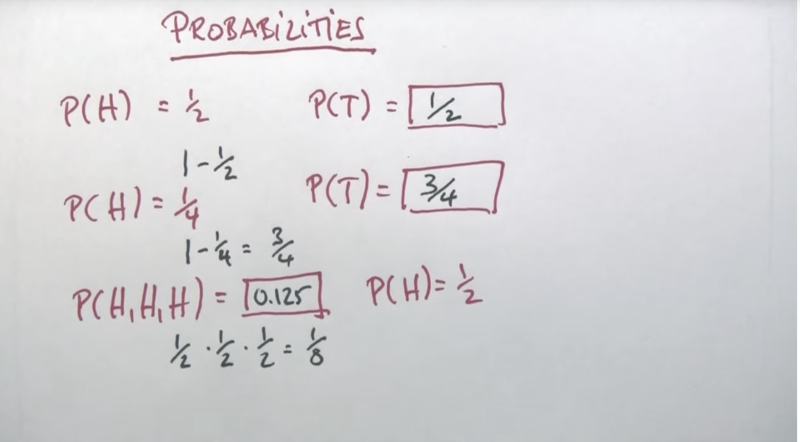• P(T) = 1 - P(H)
• since H and T are independent events, P(H,H,H) = P(H) x P(H) x P(H)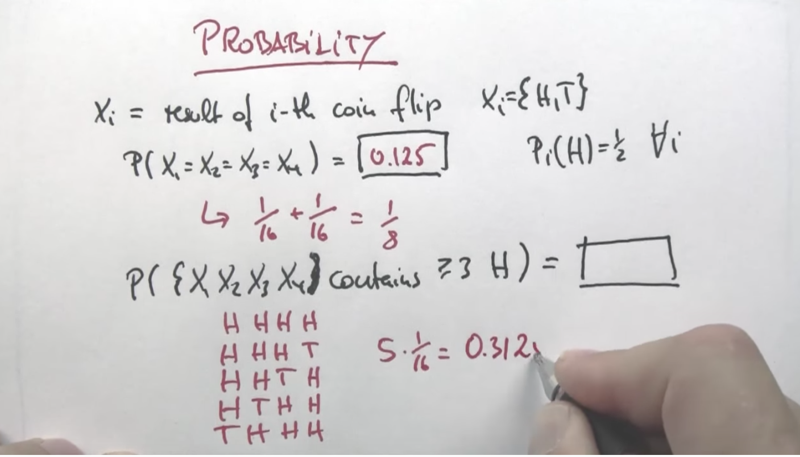• remember, P(H) and P(T) are independent

## Summary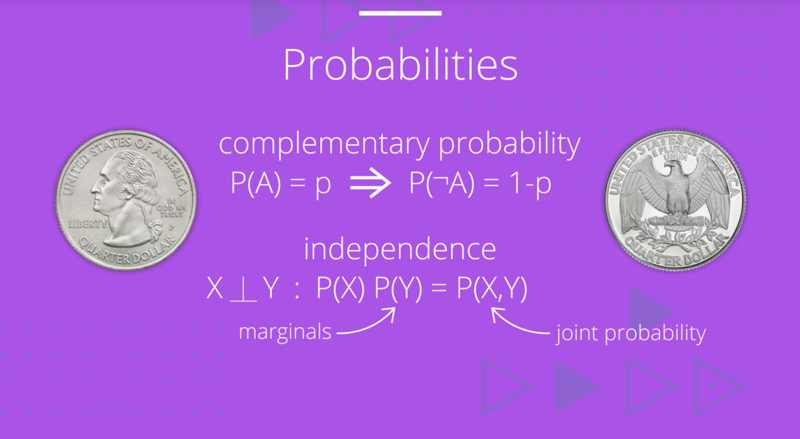# Dependence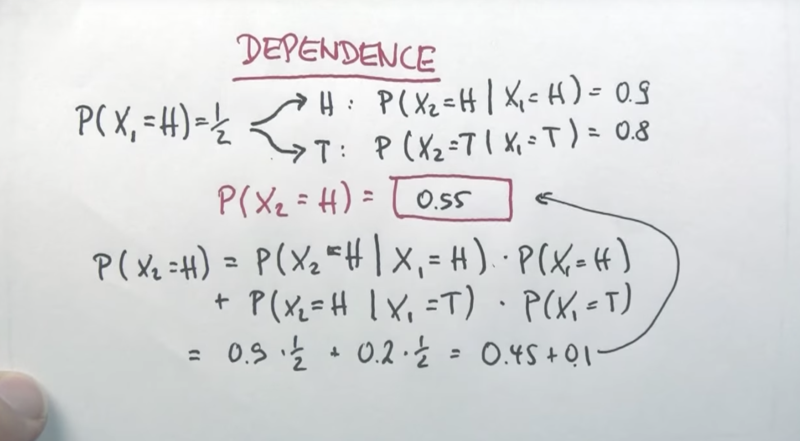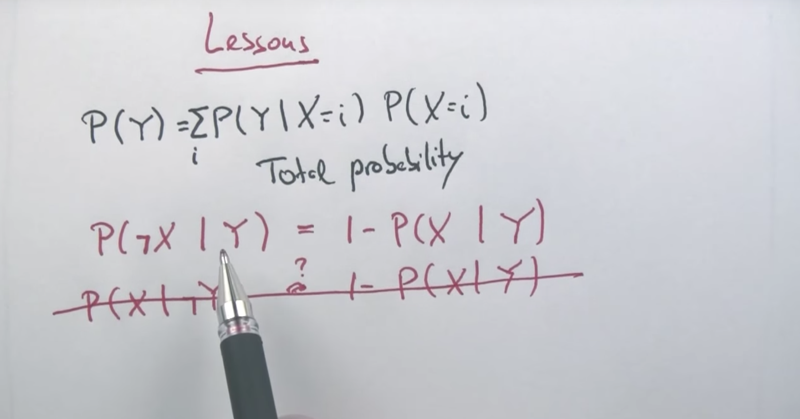# quiz: Weather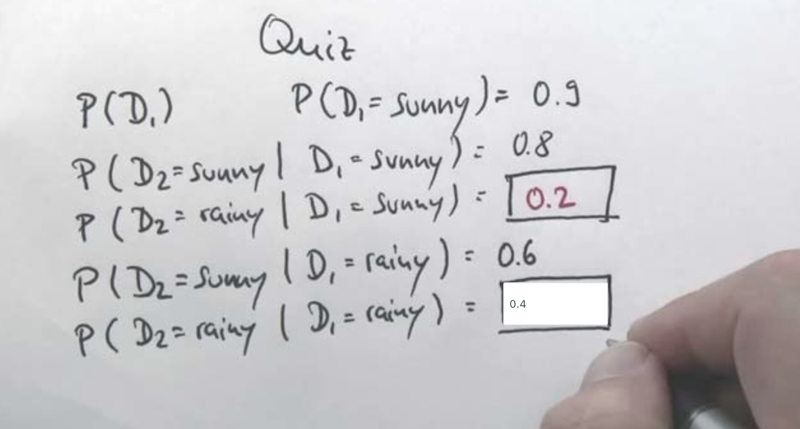• complementary rule applies for the first 2 quiz questions.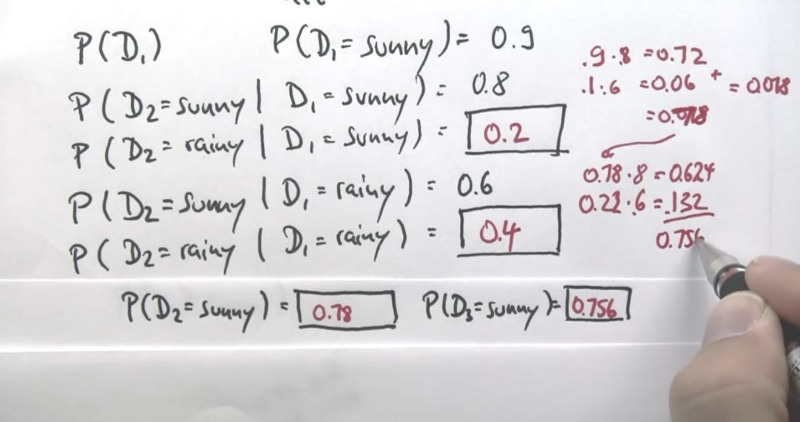• dependence rule applies to the calculation of P(D2 = Sunny) and P(D3 = Sunny)
•  P(D2 = Sunny) = P(D2 = Sunny D1 = Sunny) x P(D1 = Sunny) + P(D2 = Sunny D1 = Rainy) x P(D1 = Rainy)
•  Simillarly, * P(D3 = Sunny) = P(D3 = Sunny D2 = Sunny) x P(D2 = Sunny) + P(D3 = Sunny D2 = Rainy) x P(D2 = Rainy)

## Quiz: Cancer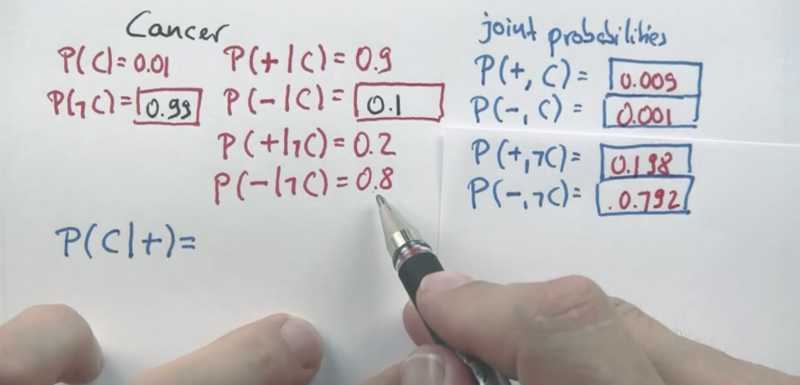• joint probability of a and b is P(a, b) = P(a) x P(b)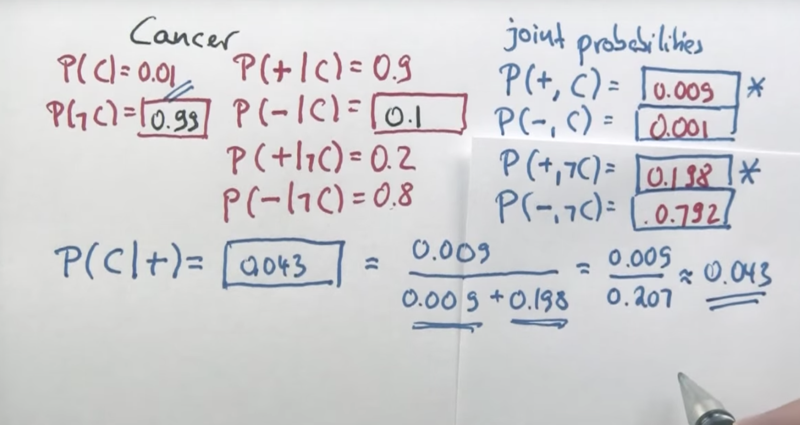•  P(C +) = P( +,C) /(P(+,C) + P(-,C))
• this is the Bayes rule!

# Bayes Rule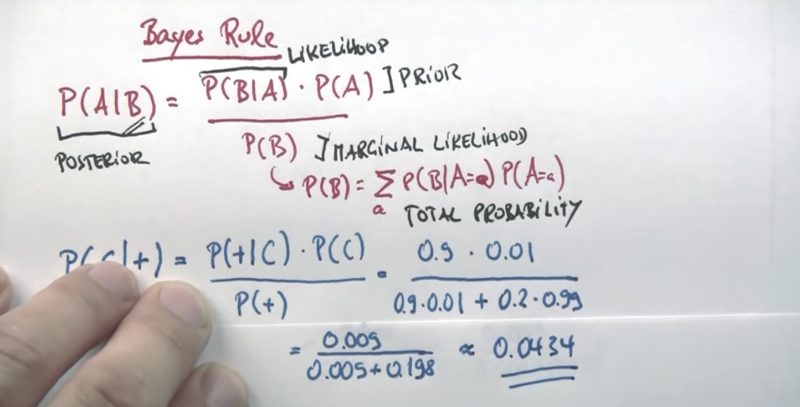• Prior
• Posterior
• Likelihood
• Marginal likelihood (Total probability)
``````20171006 初稿
``````